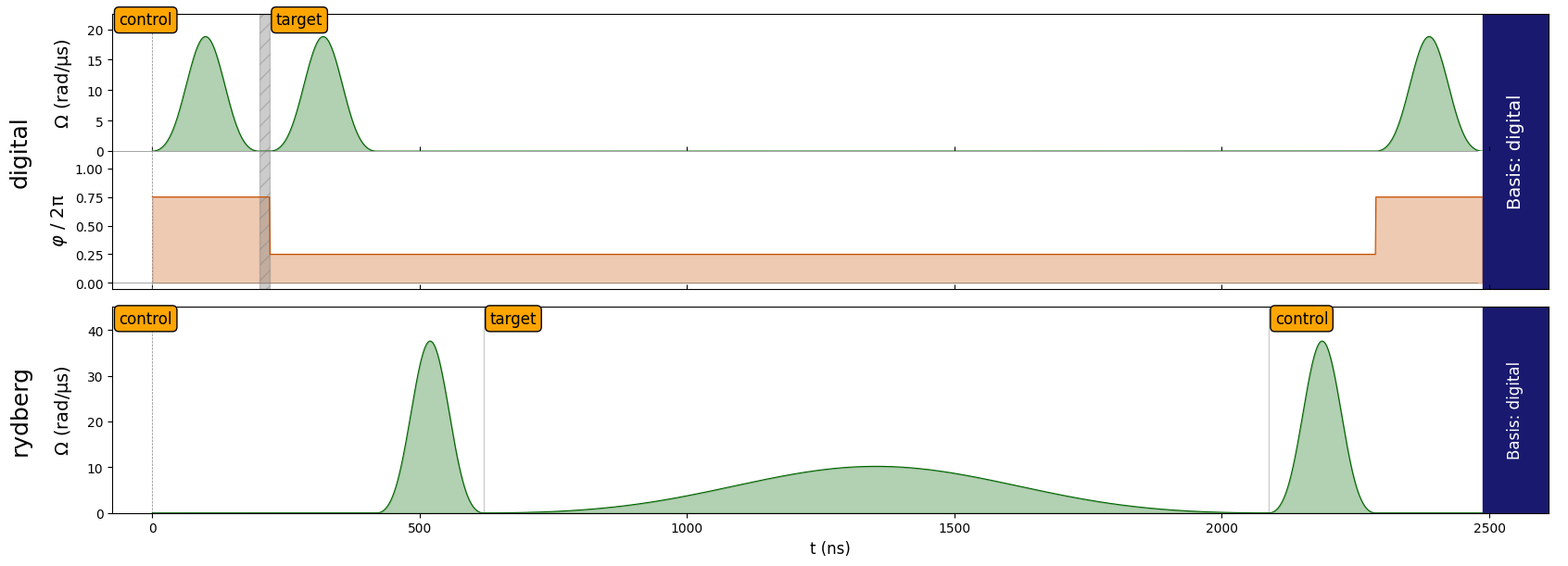# JSON Serialization

:

import numpy as np
from pulser import Pulse, Sequence, Register
from pulser.waveforms import BlackmanWaveform
from pulser.devices import DigitalAnalogDevice


Often times, it is useful to import/export a given Sequence between different locations. To enable this, the Sequence object supports serialization and deserialization into JSON-formatted strings. This will work for any given Sequence. Take for example, this sequence that creates the Bell state $$|\Phi^+\rangle = \frac{|00\rangle + |11\rangle}{\sqrt{2}}$$:

:

qubits = {"control": (-2, 0), "target": (2, 0)}
reg = Register(qubits)

seq = Sequence(reg, DigitalAnalogDevice)
pulse_time = seq.declare_variable("pulse_time", dtype=int)
seq.declare_channel("digital", "raman_local", initial_target="control")
seq.declare_channel("rydberg", "rydberg_local", initial_target="control")

half_pi_wf = BlackmanWaveform(pulse_time, area=np.pi / 2)

ry = Pulse.ConstantDetuning(amplitude=half_pi_wf, detuning=0, phase=-np.pi / 2)
ry_dag = Pulse.ConstantDetuning(
amplitude=half_pi_wf, detuning=0, phase=np.pi / 2
)

seq.target("target", "digital")

pi_wf = BlackmanWaveform(pulse_time, np.pi)
pi_pulse = Pulse.ConstantDetuning(pi_wf, 0, 0)

two_pi_wf = BlackmanWaveform.from_max_val(max_val, 2 * np.pi)
two_pi_pulse = Pulse.ConstantDetuning(two_pi_wf, 0, 0)

seq.align("digital", "rydberg")
seq.target("target", "rydberg")
seq.target("control", "rydberg")

seq.align("digital", "rydberg")
seq.measure("digital")
seq1 = seq.build(pulse_time=200)
seq1.draw()## Serialize

To serialize, use Sequence.to_abstract_repr():

:

s_readable = seq.to_abstract_repr(
json_dumps_options={"indent": 1},
seq_name="Sequence_with_defaults",
)

{
"version": "1",
"name": "Sequence_with_defaults",
"register": [
{
"name": "control",
"x": -2.0,
"y": 0.0
},
{
"name": "target",
"x": 2.0,
"y": 0.0
}
],
"channels": {
"digital": "raman_local",
"rydberg": "rydberg_local"
},
"variables": {
"pulse_time": {
"type": "int",
"value": [
0
]
}
},
"ope ...


We can note that it is possible to provide optional parameters of json.dumps such as indent via a dictionnary in the argument json_dumps_options.

Providing optional arguments to to_abstract_repr defines default parameters in the JSON object (like the name of the sequence seq_name). This does not change the Sequence object in itself, as we’ll see in the following part about deserialization.

## Deserialize

The generated strings contain all the necessary information for recreating the original sequence elsewhere (it could, for example, be saved to a file and then imported). To recover the sequence seq from s_readable (converted into JSON using Sequence.to_abstract_repr()), one should use Sequence.from_abstract_repr().

:

recovered_seq = Sequence.from_abstract_repr(s_readable)
recovered_seq.build(pulse_time=200).draw()